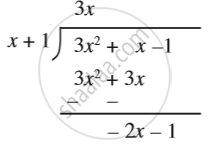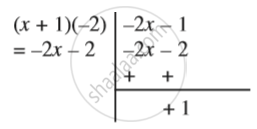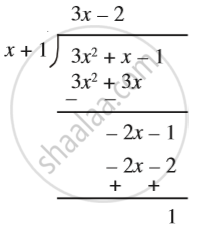# Remainder Theorem

## Notes

Let us consider two numbers 15 and 6. You know that when we divide 15 by 6, we get the quotient 2 and remainder 3. We write 15 as 15 = (6 × 2) + 3
Now let us consider an example to see how we can divide a polynomial by any non-zero polynomial.
Divide p(x) by g(x), where p(x) = x + 3x^2 – 1 and g(x) = 1 + x.
Sol-  The process of division by means of the following steps:

Step 1 : We write the dividend x + 3x^2 – 1 and the divisor 1 + x in the standard form, i.e., after arranging the terms in the descending order of their degrees. So, the dividend is 3x^2 + x –1 and divisor is x + 1.

Step 2 : We divide the first term of the dividend by the first term of the divisor, i.e., we divide 3x^2 by x, and get 3x. This gives us the first term of the quotient.
(3x^2)/x = 3x = first term of quotient

Step 3 : We multiply the divisor by the first term of the quotient, and subtract this product from the dividend, i.e., we multiply x + 1 by 3x and subtract the product 3x^2 + 3x from the dividend 3x^2 + x – 1. This gives us the remainder as –2x – 1. Fig.Step 4 : We treat the remainder –2x – 1 as the new dividend. The divisor remains the same. We repeat Step 2 to get the next term of the quotient, i.e., we divide the first term – 2x of the (new) dividend by the first term x of the divisor and obtain – 2. Thus, – 2 is the second term in the quotient.
(–2x)/x  = – 2
= second term of quotient
New Quotient = 3x – 2

Step 5 : We multiply the divisor by the second term of the quotient and subtract the product from the dividend. That is, we multiply x + 1 by – 2 and subtract the product – 2x – 2 from the dividend – 2x – 1. This gives us 1 as the remainder. Fig.This process continues till the remainder is 0 or the degree of the new dividend is less than the degree of the divisor. At this stage, this new dividend becomes the remainder and the sum of the quotients gives us the whole quotient.
Step 6 : Thus, the quotient in full is 3x – 2 and the remainder is 1.Fig.Dividend = (Divisor × Quotient) + Remainder
i.e.  3x^2 + x – 1 = (x + 1) (3x – 2) + 1

## Theorem

: Let p(x) be any polynomial of degree greater than or equal to one and let a be any real number. If p(x) is divided by the linear polynomial x – a, then the remainder is p(a).

Proof : Let p(x) be any polynomial with degree greater than or equal to 1. Suppose that when p(x) is divided by x – a, the quotient is q(x) and the remainder is r(x), i.e.,
p(x) = (x – a) q(x) + r(x)
Since the degree of x – a is 1 and the degree of r(x) is less than the degree of x – a, the degree of r(x) = 0. This means that r(x) is a constant, say r.
So, for every value of x, r(x) = r.
Therefore, p(x) = (x – a) q(x) + r
In particular, if x = a, this equation gives us
p(a) = (a – a) q(a) + r   = r,
which proves the theorem.

If you would like to contribute notes or other learning material, please submit them using the button below.

#### Video Tutorials

We have provided more than 1 series of video tutorials for some topics to help you get a better understanding of the topic.

Series 1

Series 2

### Shaalaa.com

Remainder Theorem Part 1 [00:05:26]
S
0%